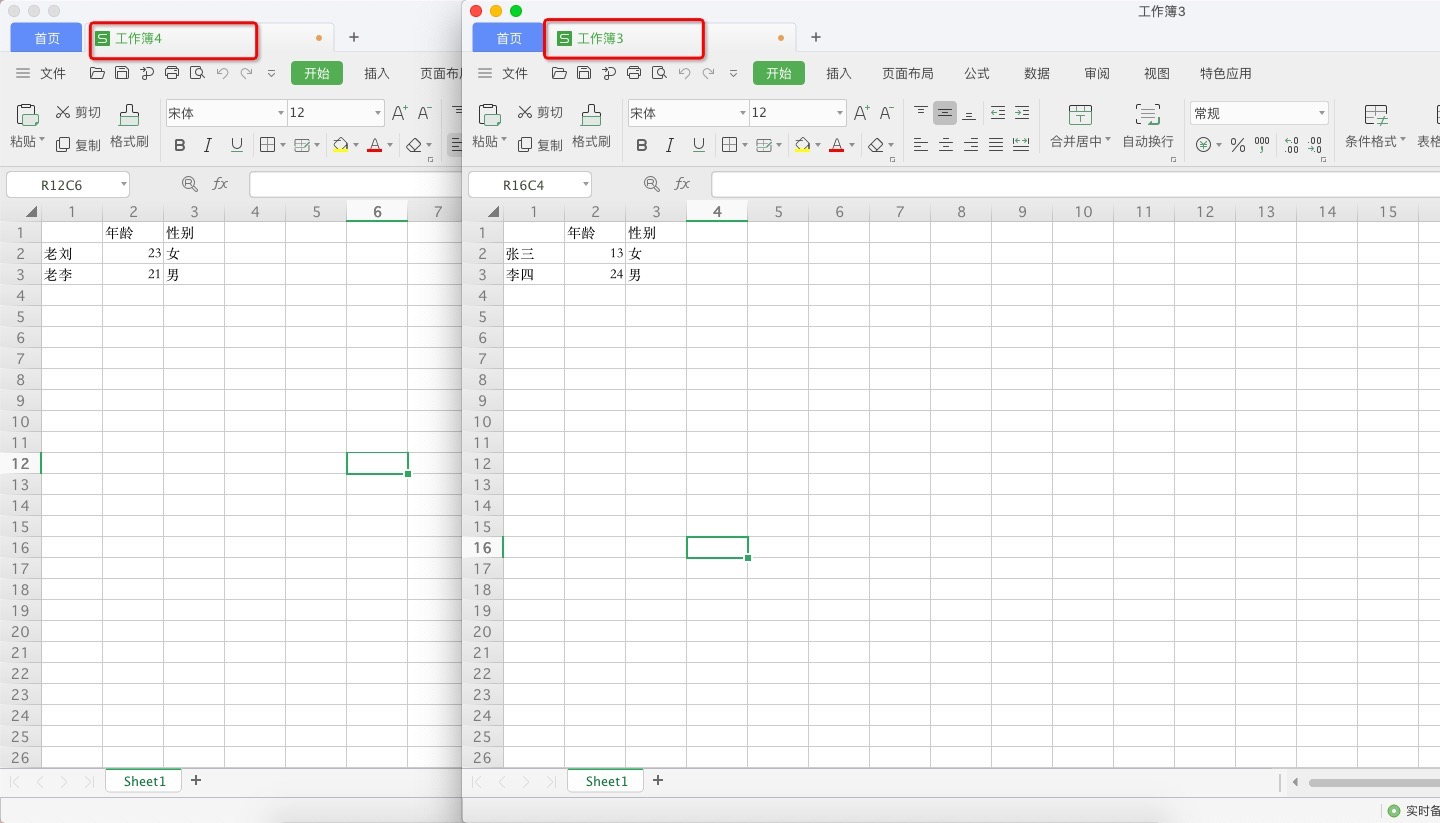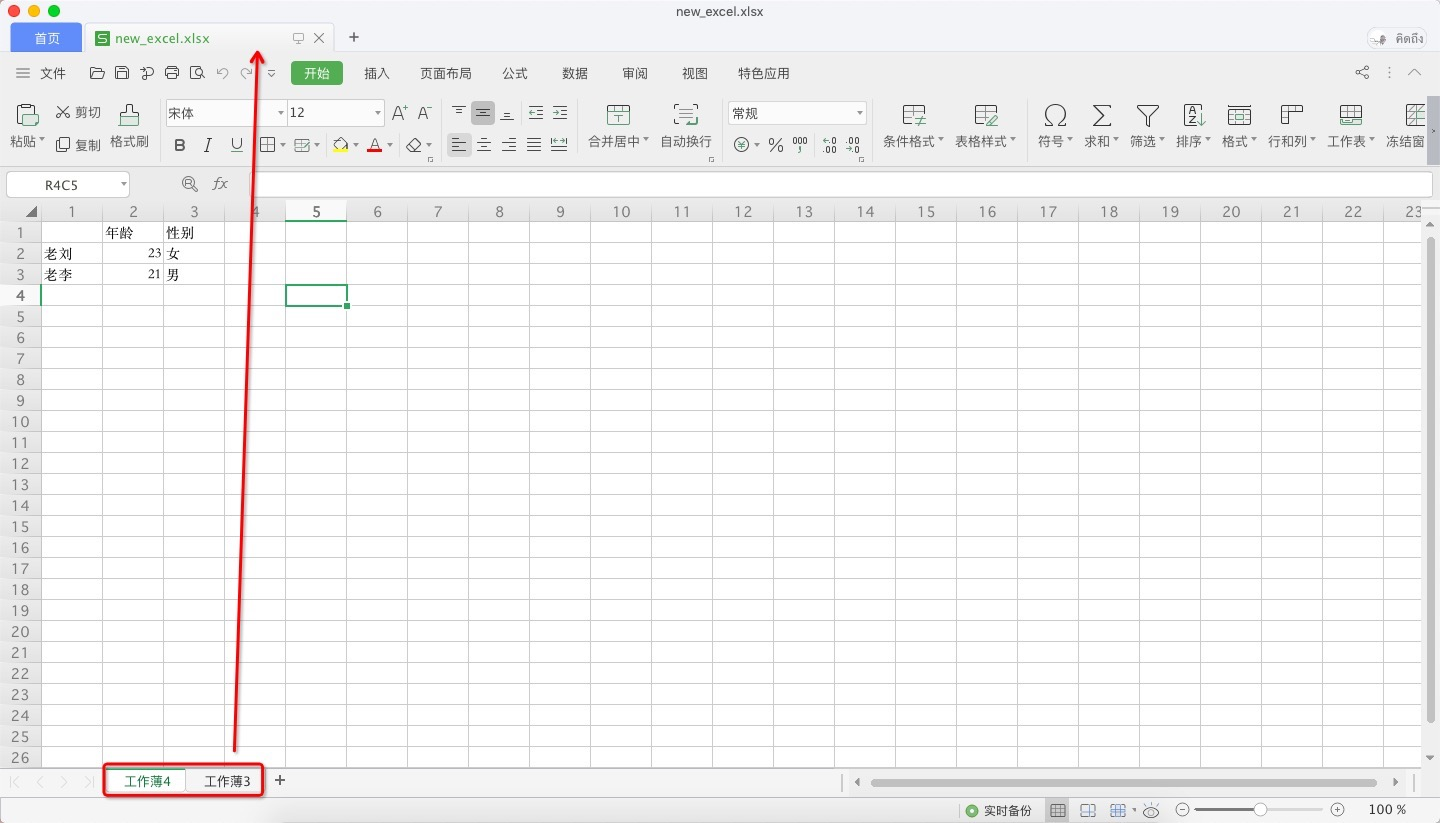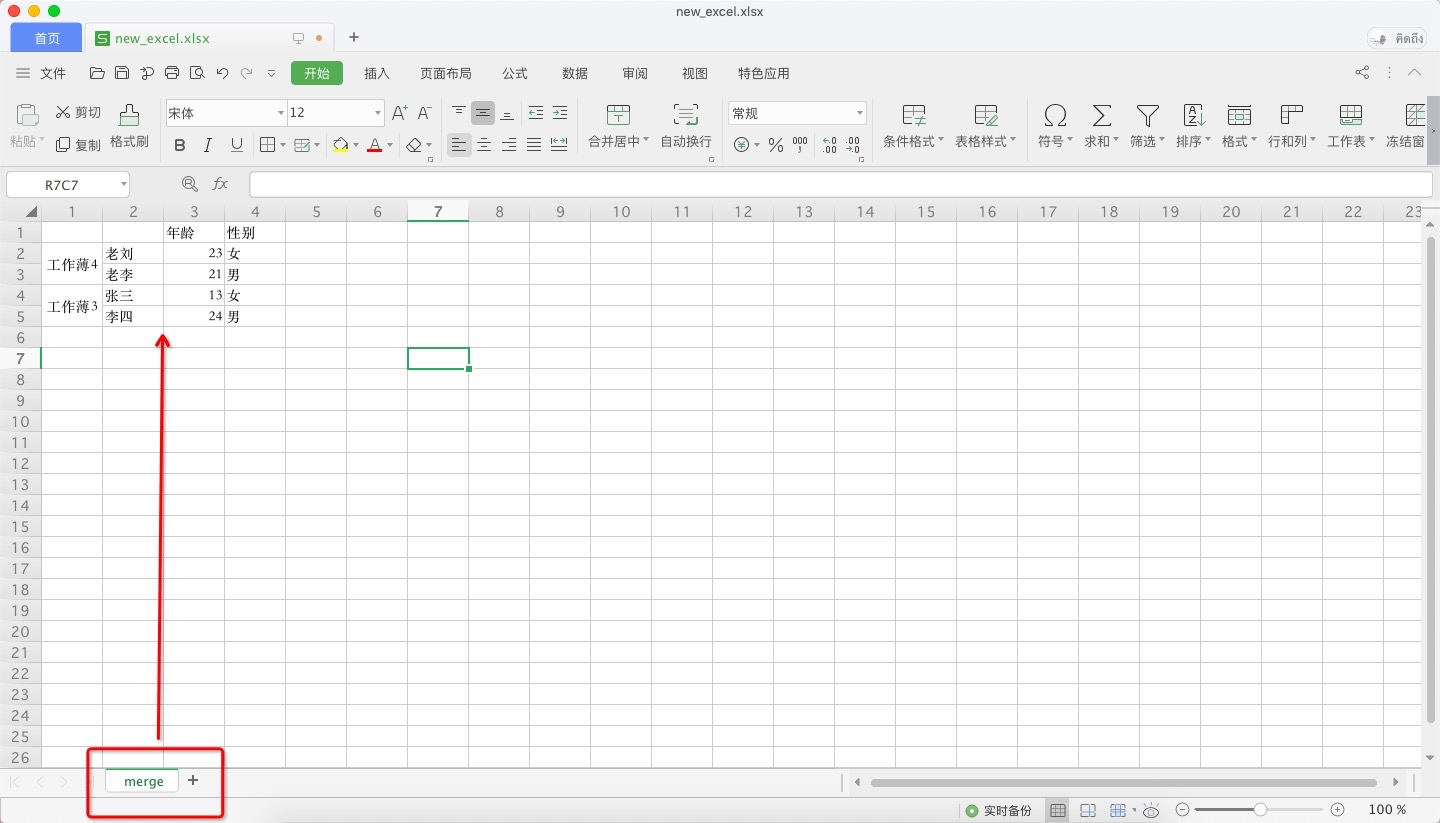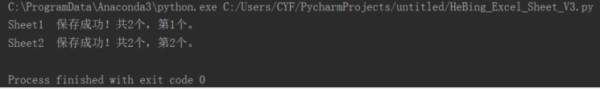• 今天小编就为大家分享一篇python 实现读取一个excel多个sheet表并合并的方法，具有很好的参考价值，希望对大家有所帮助。一起跟随小编过来看看吧
• 就是找出每一个excel中特定的sheet，把这些sheet的特定列读取出来合并到一个sheet中。 因为每一个sheet的数据都不太一样，所以稍微麻烦一点，下面使用openpyxl方式和pandas两种方式来处理。 openpyxl方式 使用...


文章目录
简介openpyxl方式pandas方式

简介
因为，每天都会有的大量excel报表汇总处理任务，所以写了一个脚本来处理。
就是找出每一个excel中特定的sheet，把这些sheet的特定列读取出来合并到一个sheet中。
因为每一个sheet的数据都不太一样，所以稍微麻烦一点，下面使用openpyxl方式和pandas两种方式来处理。
openpyxl方式
使用openpyxl方式要自己实现合并逻辑，要麻烦一些。值得注意的是，在excel中可能有公式，读取excel的时候可以使用下面的方式：

使用data_only=True,就可以得到公式计算之后的值，而不是公式本身，因为公式本身合并在另一个sheet中，公式可能就无效，甚至不对了。
下面给一个示例代码，仅供参考：
"""
pip install openpyxl
"""
from openpyxl import Workbook
import os
import re

# 模板文件
TEMPLATE_FILE = r'H:\合并\合并模板.xlsx'
# 合并结果文件
RESULT_FILE = r'H:\合并\结果.xlsx'
# 数据文件目录
DATA_ROOT_DIR = r"H:\合并"

# 文件名称正则
DATA_FILE_REG = r"(.*?)-合同导入台账\d{8}.xlsx"

# 获取要处理的文件
def get_deal_file_map():
file_sn_map = {}
fs = os.listdir(DATA_ROOT_DIR)
for f in fs:
match = re.match(DATA_FILE_REG, f)
if match:
city = match.group(1)
sn = 2
if city == '成都':
sn = 4
elif city == '杭州':
sn = 3
file_sn_map[os.path.join(DATA_ROOT_DIR, f)] = sn
return file_sn_map

# 规范化列名
def get_normal_column_name(origin_col_name):
if origin_col_name:
start = origin_col_name.find("（")
if start == -1:
return origin_col_name.strip()
else:
return origin_col_name[0:start].strip()

# 获取列名与列坐标的映射
def get_col_name_coordinate_map(sheet_row):
name_coor_map = {}
for cell in sheet_row:
# name_coor_map[get_normal_column_name(cell.value)] = cell.column_letter
name_coor_map[get_normal_column_name(cell.value)] = cell.column
return name_coor_map

# 获取模板文件的列名与列坐标映射
def get_template_name_coordinate_map(template_file_path):
table = template_wbook[template_wbook.sheetnames]
row = table[1:1]
return get_col_name_coordinate_map(row)

def deal_data_content():
"""
合并文件内容
"""
dfile_sn_map = get_deal_file_map()
save_book = Workbook()
wsheet = save_book.active
wsheet.title = 'merge-data'
tmp_col_coor_map = get_template_name_coordinate_map(TEMPLATE_FILE)
wsheet.append(list(tmp_col_coor_map.keys()))
line = 2
for data_file_path in dfile_sn_map.keys():
sheet_num = dfile_sn_map[data_file_path]

names = wbook.sheetnames

for i in range(0, sheet_num):
table = wbook[names[i]]
row = table[1:1]
data_col_coor_map = get_col_name_coordinate_map(row)
use_col = data_col_coor_map.keys() & tmp_col_coor_map.keys()
for row in table.iter_rows(min_row=2, values_only=True):
rcol_index = data_col_coor_map['城市']
city = row[rcol_index - 1]
if (city is None) or len(city.strip()) == 0:
continue
for col_name in use_col:
rcol_index = data_col_coor_map[col_name]
wcol_index = tmp_col_coor_map[col_name]
wsheet.cell(line, wcol_index, row[rcol_index - 1])
line += 1
save_book.save(RESULT_FILE)

if __name__ == '__main__':
deal_data_content()

pandas方式
相比于直接使用openpyxl的方式，使用pandas就方便多了，直接使用concat方法就可以了。
pd.concat(objs, axis=0, join='outer', join_axes=None, ignore_index=False,keys=None, levels=None, names=None,verify_integrity=False,copy=True)

参数含义
参数含义objskist，Series、DataFrame、Panelaxis默认为0，按行连接joininner、outer，默认为"outer"keyslist，最外层构建层次索引，如果为多索引，使用元组levelslist，用于构建MultiIndex的特定级别nameslist，结果层次索引中的级别的名称copyboolean，默认True。如果为False，请勿不必要地复制数据join_axes将要废弃，推荐在结果集上使用reindexignore_indexboolean，默认False。如果为True，忽略索引verify_integrityboolean，默认False。检查新连接的轴是否包含重复项
下面直接看示例：
# coding:utf-8
import pandas as pd

# 读取指定文件的指定sheet

# 按行拼接
data = pd.concat([df1, df2, df3, df4, df5], sort=False, ignore_index=True)

# 选择需要的列
header = ['日期', '合同号', '城市', '姓名', 'charge']

# 将结果写到值得excel文件
data.to_excel(r'H:\merge\result.xlsx', index=False)

主要是读取excel文件，关于pandas文件读写，可以参考：pandas读写文件
除了使用concat方法，还可以使用append方法，append方式是一个特殊的concat方法，就是concat的参数axis=0的情况，也是concat方法的axis的默认值。
既然使用了pandas，当然也可以顺便完成一些数据过滤、填充、转换之类的操作。
展开全文openpyxl pandas
• # 其中每个文件中有多个sheet，需要将其全部合并 import xlrd,xlsxwriter #设置要合并的所有文件 allxls=["/Users/xubin/myapp/pythonfile/第一个测试文件.xlsx","/Users/xubin/myapp/pythonfil...


#用python实现将三个excel合并成一个excel
#第一个测试文件 第二个测试文件 第三个测试文件
# 其中每个文件中有多个sheet，需要将其全部合并

import xlrd,xlsxwriter

#设置要合并的所有文件
allxls=["/Users/xubin/myapp/pythonfile/第一个测试文件.xlsx","/Users/xubin/myapp/pythonfile/第二个测试文件.xlsx","/Users/xubin/myapp/pythonfile/第三个测试文件.xlsx"]
#设置合并到的文件
endxls ="/Users/xubin/myapp/pythonfile/endxls.xlsx"

#打开表格
def open_xls(file):
try:
fh=xlrd.open_workbook(file)
return fh
except Exception as e:
print(str("打开出错，错误为："+e))

#获取所有sheet
def getsheet(fh):
return fh.sheets()

#读取某个sheet的行数
def getnrows(fh,sheet):
table=fh.sheets()[sheet]
content=table.nrows
return content

#读取某个文件的内容并返回所有行的值
def getfilect(fh,fl,shnum):
fh=open_xls(fl)
table=fh.sheet_by_name(shname[shnum])
num=getnrows(fh,shnum)
lenrvalue=len(rvalue)
for row in range(0,num):
rdata=table.row_values(row)
rvalue.append(rdata)
print(rvalue[lenrvalue:])
filevalue.append(rvalue[lenrvalue:])
return filevalue

#存储所有读取的结果
filevalue=[]
#存储一个标签的结果
svalue=[]
#存储一行结果
rvalue=[]
#存储各sheet名
shname=[]

#读取第一个待读文件，获得sheet数
fh=open_xls(allxls)
sh=getsheet(fh)
x=0
for sheet in sh:
shname.append(sheet.name)
svalue.append([])
x+=1
#依次读取各sheet的内容
#依次读取各文件当前sheet的内容
for shnum in range(0,x):
for fl in allxls:
print("正在读取文件："+str(fl)+"的第"+str(shnum)+"个标签的…")
filevalue=getfilect(fh,fl,shnum)
svalue[shnum].append(filevalue)
#print(svalue)
#print(svalue)
#由于apped具有叠加关系，分析可得所有信息均在svalue中存储
#svalue元素数量为sheet标签数(sn)*文件数(fn)
sn=x
fn=len(allxls)
endvalue=[]

#设置一个函数专门获取svalue里面的数据，即获取各项标签的数据
def getsvalue(k):
for z in range(k,k+fn):
endvalue.append(svalue[z])
return endvalue

#打开最终写入的文件
wb1=xlsxwriter.Workbook(endxls)
#创建一个sheet工作对象
polit=0
linenum=0
#依次遍历每个sheet中的数据
for s in range(0,sn*fn,fn):
thisvalue=getsvalue(s)
tvalue=thisvalue[polit:]
#将一个标签的内容写入新文件中
for a in range(0,len(tvalue)):
for b in range(0,len(tvalue[a])):
for c in range(0,len(tvalue[a][b])):
#print(linenum)
#print(c)
data=tvalue[a][b][c]
ws.write(linenum,c,data)
linenum+=1
#叠加关系，需要设置分割点
polit=len(thisvalue)
wb1.close()
123456789101112131415161718192021222324252627282930313233343536373839404142434445464748495051525354555657585960616263646566676869707172737475767778798081828384858687888990919293949596979899100101102


展开全文• 最近有两个需求，第一是要求把指定目录下多个结构相同的excel合并为一个excel，每个excel都对应合并后的一个sheet；第二是要求把指定目录下多个结构相同的excel合并为一个sheet，并在合并后的sheet添加一列，标识...
最近有两个需求，第一是要求把指定目录下多个结构相同的excel合并为一个excel，每个excel都对应合并后的一个sheet；第二是要求把指定目录下多个结构相同的excel合并为一个sheet，并在合并后的sheet添加一列，标识数据来源与哪一个sheet，来自同一数据源的多行数据第一列要求合并单元格。
多个excel合并的效果：import os
import pandas as pd
import xlwt

import xlrd
from xlutils.copy import copy
import sys

# 要写入的excel全路径及名称
file_to = sys.argv
# 要合并的excel的目录
rootdir = sys.argv
# 要合并的excel文件名的公共部分
file_name = sys.argv

# 新建列表，存放文件名
filename_excel = []

# 列出文件夹下所有的目录与文件
def list_all_files(rootdir):
_files = []
list = os.listdir(rootdir)
for i in range(0, len(list)):
path = os.path.join(rootdir, list[i])
if os.path.isdir(path):
_files.extend(list_all_files(path))
if os.path.isfile(path):
_files.append(path)
return _files

# 获取指定的文件名和要写入的sheet名
def get_file(files, file_name):
# 存放该excel的list
file_list = []
# 存放文件去除后缀名的list
file_name_list = []
for file in files:
# 判断此文件名称是否包含此关键名称
if file_name in file:
file_list.append(file)
print(file)
# 截取要写入的sheet名（此处截取的规则是：截取最后一个'/'之后，'.'之前，'）'之后）
# 结案、提出抗诉（件）（温州2018年12月-2019年11月）
file_name_list.append(file.split('/')[-1].split('.').split(')'))
return file_list, file_name_list

def merge_files(file_to, files, file_names):
# 创建sheet
workbook = xlwt.Workbook()
for file_name in file_names:
# print(file_name)
# print(files[file_names.index(file_name)])
workbook.save(file_to)

# 向不同sheet写入数据
writer = pd.ExcelWriter(file_to)
for file_name in file_names:
# print(df.columns)
# df.rename(columns={'Unnamed: 0': ''})
df.to_excel(writer, sheet_name=file_name, startcol=0, index=False)
writer.save()

rb = xlrd.open_workbook(file_to, formatting_info=True)
wb = copy(rb)
# 修改第一个cell的值('Unnamed: 0' -> '')   这个步骤可根据实际情况选择
for file_name in file_names:
ws = wb.get_sheet(file_name)
ws.write(0, 0, '')
wb.save(file_to)

files, file_names = get_file(list_all_files(rootdir), file_name)
merge_files(file_to, files, file_names)


多个sheet合并成一个sheet的效果：import os
import pandas as pd
import sys

# # 要写入的excel全路径及名称
# file_to = sys.argv
# # 要合并的excel的目录
# rootdir = sys.argv
# # 要合并的excel文件名的标识
# file_name = sys.argv

# 新建列表，存放文件名
filename_excel = []

# 列出文件夹下所有的目录与文件
def list_all_files(rootdir):
_files = []
list = os.listdir(rootdir)
for i in range(0, len(list)):
path = os.path.join(rootdir, list[i])
if os.path.isdir(path):
_files.extend(list_all_files(path))
if os.path.isfile(path):
_files.append(path)
return _files

# 获取指定的文件
def get_file(files, file_name_list):
file_list = []

for file_name in files:
flag = 1
# 判断此文件名称是否包含此关键名称
for i in file_name_list:
if i not in file_name:
flag = 0
if flag == 1:
file_list.append(file_name)
return file_list

# for i in list:
#     print(i)

#  合并第一列单元格
def merge_cell(result_file, sheet_name):
ws = wb[sheet_name]

# 获取第一列数据
type_list = []
i = 2
while True:
r = ws.cell(i, 1).value
if r:
type_list.append(r)
else:
break
i += 1

# 判断合并单元格的始末位置
s = 0
e = 0
flag = type_list
for i in range(len(type_list)):
if type_list[i] != flag:
flag = type_list[i]
e = i - 1
if e >= s:
ws.merge_cells("A" + str(s + 2) + ":A" + str(e + 2))
s = e + 1
if i == len(type_list) - 1:
e = i
ws.merge_cells("A" + str(s + 2) + ":A" + str(e + 2))
wb.save(result_file)

def merge_files(file_list, file_to):
frames = []
for i in file_list:
index_list = []
for num in range(0, df.shape):
# 可根据实际需求获取第一列的字段名称
index_list.append(i.split('/')[-1].split('.'))

# 在第一列插入数据来源文件名
df.insert(0, '文件', index_list)
filename_excel.append(i)
frames.append(df)

# 合并所有数据
result = pd.concat(frames, axis=0)
result.to_excel(file_to, sheet_name='merge')

# 去掉第一列无用数据
ws = wb.active
ws.delete_cols(1)
wb.save(file_to)

# 按照第一列合并相同单元格
merge_cell(file_to, 'merge')

# 修改此时第一行第二列cell的值('Unnamed: 0' -> '')
rb = xlrd.open_workbook(file_to)
wb = copy(rb)
ws = wb.get_sheet('merge')
ws.write(0, 1, '')
wb.save(file_to)

list = get_file(list_all_files(rootdir), file_name)
merge_files(list, file_to)
os.rename(file_to, file_to[:-1])

展开全文• 主要为大家详细介绍了Python多个excel文件合并为一个文件，具有一定的参考价值，感兴趣的小伙伴们可以参考一下
• 前两天给大家分享了Python自动化文章：手把手教你利用Python轻松拆分Excel多个CSV文件，而后在Python进阶交流群里边有读者遇到一个问题，他有很多个Excel表格，他需要对这些Excel文件进行合并。 一、前言 ...
大家好，我是崔艳飞。前两天给大家分享了Python自动化文章：手把手教你利用Python轻松拆分Excel为多个CSV文件，而后在Python进阶交流群里边有读者遇到一个问题，他有很多个Excel表格，他需要对这些Excel文件进行合并。一、前言
大家好，我是崔艳飞。前两天给大家分享了Python自动化文章：手把手教你利用Python轻松拆分Excel为多个CSV文件，而后在Python进阶交流群里边有读者遇到一个问题，他有很多个Excel表格，他需要对这些Excel文件进行合并。
诚然，一个一个打开复制粘贴固然可行，但是该方法费时费力，还容易出错，几个文件还可以手动处理，要是几十个甚至上百个，你就抓瞎了，不过这问题对Python来说，so easy，一起来看看吧!
二、项目目标
用Python实现多Excel、多Sheet的合并处理。
三、项目准备
软件：PyCharm
需要的库：pandas， xlrd，os
四、项目分析
1)如何选择要合并的Excel文件?
利用os，获取所有要合并的Excel文件。
2)如何选择要合并的Sheet?
利用xlrd库进行Excel读取，获取要合并的Sheet名。
3)如何合并?
利用pandas库，对所有Sheet名逐一循环打开，通过concat()进行数据追加合并即可。
4)如何保存文件?
利用to_excel保存数据，得到最终合并后的目标文件。
五、项目实现
1、第一步导入需要的库
import pandas as pd
import xlrd
import os

2、第二步选择要合并的Excel文件
#要合并文件路径 path="D:/b/" #获取文件夹下所有EXCEL名 xlsx_names = [x for x in os.listdir(path) if x.endswith(".xlsx")]
3、第三步选择要合并的Sheet
# 获取第一个EXCEL名
xlsx_names1 = xlsx_names
aa = path + xlsx_names1
#打开第一个EXCEL
first_file_fh=xlrd.open_workbook(aa)
# 获取SHEET名
first_file_sheet=first_file_fh.sheets()

4、第四步对Sheet内容进行循环合并
#按SHEET名循环
for sheet_name in sheet_names:
df = None
# 按EXCEL名循环
for xlsx_name in xlsx_names:
sheet_na = pd.ExcelFile(path + xlsx_name).sheet_names
if sheet_name in sheet_na:
#print(sheet_name)
if df is None:
df = _df
else:
df = pd.concat([df, _df], ignore_index=True)
else:continue

5、第五步保存合并后的文件
df.to_excel(excel_writer=writer, sheet_name=sheet_name, encoding="utf-8", index=False)
print(sheet_name + "  保存成功！共%d个，第%d个。" % (len(sheet_names),num))
num += 1
writer.save()
writer.close()

六、效果展示
1、处理前Excel数据：2、运行进度提示：3、合并后的结果：七、总结
本文介绍了如何利用Python对多个Excel文件、多Sheet进行合并处理，减少了很多复制粘贴的麻烦，省时省力，还不容易出错，代码不多，循环追加有点绕，想想也就明白了，不懂的随时留言提问，大家一起学习进步。
有想法的小伙伴还可以将文章中的代码进行打包，做成一个exe可执行的小软件，包装好发给别人使用，也可以赚点小费噢，关于打包的教程这里不再赘述，欢迎前往：三个你必须要记住的Pyinstaller打包命令——利用Python实现打包exe。
八、彩蛋
下面两份代码是群内小伙伴提供了，小编也自行测试了下，亲测有效，欢迎大家积极尝试噢!
来自群友Jayson的代码：
# -*- coding: utf-8 -*-
# @Author: hebe
# @Date:   2020-04-18 18:31:03
import os
import glob
import openpyxl

def merge_xlsx_files(xlsx_files):
ws = wb.active
ws.title = "merged result"

for  filename in xlsx_files[1:]:
sheet = workbook.active
for row in sheet.iter_rows(min_row=1):
values = [cell.value for cell in row]
ws.append(values)
return wb

#path is very important here , must true.
def get_all_xlsx_files(path):
xlsx_files = glob.glob(os.path.join(r'C:\\Users\\pdcfi\\Desktop\\', '*.xlsx'))
sorted(xlsx_files, key=str.lower)
return xlsx_files

def main():
xlsx_files = get_all_xlsx_files(os.path.expanduser('~lmx'))
wb = merge_xlsx_files(xlsx_files)
wb.save('merged_form.xlsx')

if __name__ == '__main__':
main()

print("all excel append OK!")

来自好友刘早起的代码：
# -*- coding: utf-8 -*-
import glob

path = "C:\\Users\\pdcfi\\Desktop\\excel\\"
new_workbook = Workbook()
new_sheet = new_workbook.active

# 用flag变量明确新表是否已经添加了表头，只要添加过一次就无须重复再添加
flag = 0

for file in glob.glob(path + '/*.xlsx'):
sheet = workbook.active

coloum_A = sheet['A']
row_lst = []
for cell in coloum_A:
if cell:
print(cell.row)
row_lst.append(cell.row)

if not flag:
flag = 1

for row in row_lst:
data_lst = []
for cell in sheet[row]:
data_lst.append(cell.value)
new_sheet.append(data_lst)

new_workbook.save(path + '/' + '符合筛选条件的新表.xlsx')
来自群友Engineer的代码：

import tkinter as tk
from tkinter import filedialog
import os
import pandas as pd
import glob

root = tk.Tk()
root.withdraw()

# 选择文件夹位置
lst = []

# 读取文件夹下所有文件（xls和xlsx都读取）
for i in glob.glob(filelocation + "\\\\" + "*.*"):
if os.path.splitext(i) in [".xls", ".xlsx"]:

# 保存合并后的excel文件
filetypes=[("Excel 工作簿", "*.xlsx"),
("Excel 97-2003 工作簿", "*.xls")]))
pd.concat(lst).to_excel(writer, 'all', index=False)
writer.save()

print('\n%d个文件已经合并成功！' % len(lst))

当然了，实现本文功能不仅仅限于上面提及的3种方式，使用pandas也是可以做到的，如果你还有其他方法，欢迎交个朋友一起学习交流!
展开全文• 之前想把一个工作簿中结构相同的多个sheet进行合并，由于sheet太多，不可能手动完成，又不会VBA编程，所以就谢了一个实现此功能的python程序。希望对有相同需求的兄弟姐妹有帮助。 用到的Python模块如下： xlrd ...
• 使用Python将多个excel多个sheet合并到一个excel ##读取Excel文件 import xlrd ##将文本写入excel文件 import xlsxwriter ##读取excel文件 def getexcel(excel): f=xlrd.open_workbook(excel) return f ##获取...
• 202102 ，一个sheet 等等 目标：将多个excel文件中的所有sheet合并在同一excel文件中，并将同名sheet进行合并 因为完全没有任何相关知识，只能在网站查询成熟方法，找到方法如下，但excel文件中如果存在单个名称的...
• #用python实现将三个excel合并成一个excel #第一个测试文件 第二个测试文件 第三个测试...# 其中每个文件中有多个sheet，需要将其全部合并 import xlrd,xlsxwriter #设置要合并的所有文件 allxls=["/Users/xubin/m...
• 之前经常会碰到一个excel多个sheet文件的情况，当数据少的时候，我们可以把多个sheet文件合成一个xlxs格式的excel，但是当数据很大的时候，我们可以合成一个csv格式的文件，也便于操作。如图所示，我们可以看到有4...
• python读取excel多个sheet页并合并成一页 import xlrd import xlwt data = xlrd.open_workbook("database.xlsx") workbook = xlwt.Workbook() worksheet = workbook.add_sheet('sheets2one') '''sheet页''...
• Python合并Excel中所有的sheet源代码 首先读取Excel中所有的sheet名称，然后遍历所有的sheet，最后将每张sheet拼接起来 源代码 去博客设置页面，选择一款你喜欢的代码片高亮样式，下面展示同样高亮的 代码片. #/usr/...Python Python数据分析
• 一、背景 用python（2.7） pandas处理excel多个sheet，各sheet之间有关联字段，起到的效果相当于多张RDB的表，做联表查询。 二、da
• Python CSV 合并多个sheet工作表 2、csv合并方法 import pandas as pd analysis_file = outDir+delimiter+'analysis_result.xlsx' writer = pd.ExcelWriter(analysis_file) csv_file1 = pd.read_csv...csv
• 运营人员需要历年的订单数据，这就需要把多个文件夹下面的excel文件合并到一个sheet中，之前的解决的办法是用VBA把多个excel文件合并成一个表的多个sheet,再把多个sheet合并成一个sheet这种办法对数据量不多的可以...
• 想不断尝试的老Amy，开启了思考模式：“我要怎么实现这需求呢？”。 不用着急，首先我们来分析数据本身。 分析数据特征如下： 数据所在路径 C:\Users\logic\Desktop\mytest\file_dir data01.xlsx 数自动化办公
• 一个excel中有多个sheet,如何将多个sheet合并成同一个sheet(那几个sheet数据格式一致,比如说第一行的表头一致) 先准备数据(一个excel里有多个需要合并的sheet) ①需要合并的sheet ②每个sheet里的表头(一致的数据...
• 点击上方“Python爬虫与数据挖掘”，进行关注回复“书籍”即可获赠Python从入门到进阶共10本电子书今日鸡汤不堪玄鬓影，来对白头吟。一、前言大家好，我是崔艳飞。前两天给大家分享了Py...数据挖掘 csv 项目管理 poi
• import scipy.io as sio import pandas as pd import os excel_path='D:/Documents/Experiments/Results/MDE'#待合并excel文件的目录 ...#遍历目录下的每合并文件 for file in os.listdir(excel
• #第一步：调用pandas包 ...iris = pd.read_excel('./iris_sheets.xlsx',None)#读入数据文件 keys = list(iris.keys()) #第三步：数据合并 iris_concat = pd.DataFrame() for i in keys: iris1 = iris[i] ...数据分析 pandas
• import xlrd import pandas as pd from pandas import DataFrame ...excel_name = '%sxxx.xls' % DATA_DIR wb = xlrd.open_workbook(excel_name) # print(wb) # 获取workbook中所有的表格 sheets = wb.sh
• 参照了这篇文章，但是最后输出的时候代码报错，解决方案详细内容可见Python处理Excel遇到的“got invalid input value of type ‘xml.etree.ElementTree.Element‘＞, exp”。 下面直接把自己最后修改好的代码附上 ...
• python合并多个excel为一个excel的不同sheet和将一个excel分解为不同的sheet一、合并多个excel为一个excel的不同sheet（一）全部合并（二）部分合并二、将一个excel分解为多个 日常处理excel文件很繁琐，尤其是将...
• 以上这篇python 实现读取一个excel多个sheet表并合并的方法就是小编分享给大家的全部内容了，希望能给大家一个参考，也希望大家多多支持脚本之家。 原文链接：...
• # 每个sheet中加入一列，列内容填写sheet名 # # 循环遍历所有sheet alldata = DataFrame() for i in sheets: df = pd.read_excel(path, sheet_name=i) df.insert(0, 'sheetname', i) alldata = alldata.append(df) ...
• python合并csv、excel等文件 首先：导入所需的库import pandas as pd 其次：输入以下代码 #创建一输出文件 writer = pd.ExcelWriter('E:/Test/test.xlsx') data = pd.read_table('E:/Test/test1.csv',sep=',',...csv
• 将多个CSV文件，合并到一个Excel文件中的，多个sheet工作表。 前言 网上大多方法都是将csv直接合并在一起，也不分别创建sheet表。 还有一些解答说CSV不支持合并多个sheet表。 网上有用宏命令的，我试了，但是...
• ## python-Excel多个表格合并

万次阅读 多人点赞 2019-02-19 15:35:42
工具：python3.7.1 用到的模块：xlrd（负责读取数据） xlsxwriter（负责写入数据） glob（查找符合自己目的的文件） 一·模块安装（pip） 注意：这里我用的是pip模块来安装，部分python版本没有此功能，可自行百度 ......

# python合并excel多个sheetpython 订阅